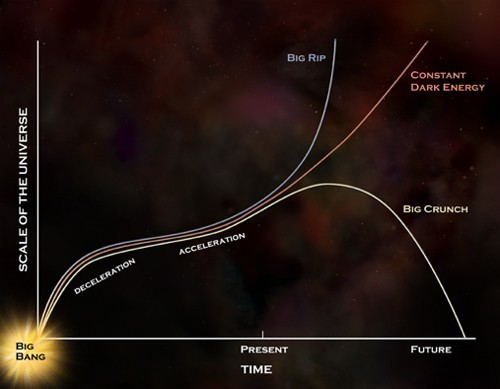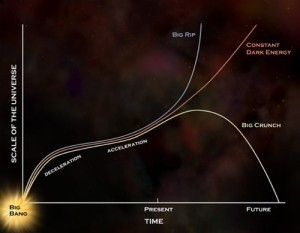# The Phantom Menace

In Dark Energy by Brian Koberlein3 Comments

We know the universe is expanding, and we know it is doing so at an ever increasing rate. This cosmic acceleration is part of the evidence for dark energy, which current observations put at about 68% of the observable universe. But beyond its existence as some kind of energy, we’re still trying to determine just what dark energy is.

The most widely accepted model for the universe, sometimes called the standard model of cosmology, is known as the ΛCDM model. The Λ or “lambda” refers to the dark energy parameter known as the cosmological constant (which often uses lambda to represent its value). The CDM stands for Cold Dark Matter, which is the type of dark matter currently best supported by observation. In the ΛCDM model, the matter of the universe consists of regular matter (about 5%), cold dark matter (about 27%). Dark energy is then caused by a cosmological constant, which is a property of space and time itself, giving rise to dark energy.

The ΛCDM model makes a couple of very important predictions about the universe that can be tested against observation. The first is the the universe is, on average, flat. This means that local masses can curve space around them (with the effect of gravitational attraction), but since the cosmological constant curves space in an opposite way (cosmic expansion), the total average should be zero. Current observations agree with this prediction, though is a hint in the data of the cosmic microwave background that the curvature might not be exactly zero, as I’ve written about before.Predictions of different cosmological models.
Credit: NASA, CXC, M. Weiss

A second prediction is about the equation of state for dark energy. This boils down to a parameter in cosmology known as w. According the the ΛCDM model, w should be exactly equal -1. If w = -1, then dark energy is a constant, and can be described in general relativity by the cosmological constant. Again, observations agree pretty well with this prediction, but a new paper summarizing observational data from Pan-STARRS seems to show that w might not be -1 after all.

Pan-STARRS (the Panoramic Survey Telescope & Rapid Response System) has been making a medium deep sky survey that has observed 112 type 1a supernovae. These supernovae observations were combined with other observations for a total of 313 type 1a supernovae. Type 1a supernovae are useful because they have a consistent maximum brightness. This mean you can observe their apparent brightness to determine how far away they are. You can also measure the redshift of their light to determine cosmic expansion. So observations of these supernovae let us determine the value of w.

What the team found was that if you just take the supernovae data, the ΛCDM model gives an experimental value for w of -1.015 give or take about 0.1 either way, which agrees with the model. But if you take the supernova data and combine it with other observational data, including the Planck observations of the Cosmic Microwave Background and observation of the Baryon Acoustic Oscillation, then you get a different value, w = -1.186 give or take 0.07. If this value is correct then the ΛCDM model isn’t an accurate description of the universe.

If the w parameter is truly less than one, then inflation cannot be due to a cosmological constant. One of the more popular models giving a w value less than -1 is known as phantom energy. This would be a particularly strong form of dark energy, and if true could mean the universe would continue to expand at an ever accelerating rate until it ended as a Big Rip.

Before you run out and tell your friends that the standard cosmological model is wrong, keep in mind that these results are tentative. They also aren’t overly strong. In any observational result there is a chance for observation bias, which would skew the results a bit. Then there is the fact that there can be random variations in the data. The reliability of an observation is often given in a statistical term known as sigma. In this case the result is at 2-sigma, which means there is a 1 in 20 chance that the deviation from w = -1 is just random variation. In science we generally set the bar at 5-sigma, which is about a 1 in 1.7 million chance of being random variation. The authors themselves note that the data isn’t strong enough to make a clear determination.

Future observations by Pan-STARRS will likely triple the number of supernovae we can use to determine w. So in time the result, whether it agrees or disagrees with ΛCDM, will reach a strong confidence level. By then we’ll know if our standard cosmological model is correct, or if it faces a phantom menace.

1.A. I’m shocked to learn that the ΛCDM model depends on a property of nature equalling exactly what our mathematics define as -1. That seems too improbably convenient. What am I missing? B. If “w” doesn’t equal -1, does that mean dark energy also is not a property of space-time as well?

2.I have a theory on how dark energy could end up reversing itself. A theory beginning with the big bang, the whole lifespan of the universe and the whole shape lifespan of the universe.
You have to imagine the whole lifespan of the universe spreads top to bottom on a sphere. Imagine the big bang starts at the north pole of the sphere. The universe then falls downwards down the sphere increasing in speed. Galaxies are moving away from each other as the head towards to equator of the sphere. I estimate our current universe is dounut shaped somewhere between the north pole and the equator of the sphere. This should explain dark energy if this is true. Once the universe passes the equator point and starts heading to the south pole, Galaxies will start to get closer together again. Becoming the opposite of dark energy. Finally heading towards the south pole in a big crunch, becoming the end of our known universe.
I see the two poles as some oppositely charged enterties. With a force we are not yet aware of.
The universe can still be flat within the universe as it moves across the sphere.

1.Author

It looks like what you’ve done is replaced “dark energy” with some force that makes it fall through the sphere. There are actually lots of ideas about an expanding then contracting universe. Currently the evidence doesn’t support them.